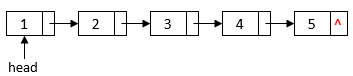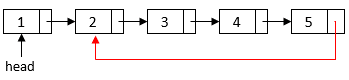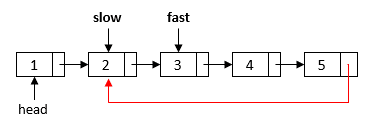## 1. Introduction

In Computer Science, a linked list is a linear data structure in which a pointer in each element determines the order.

In this tutorial, we’ll show how to check if a linked list is a circular linked list.

Each element of a linked list contains a data field to store the list data and a pointer field to point to the next element in the sequence. We can use apointer to point to the start element of a linked list:In a regular linked list, the last element has no next element. Therefore, itspointer field is. However, in a circular linked list, the last element has a reference to one of the elements in the list:## 3. Hash Table Solution

It is easy to see that if a linked list contains a cycle, we’ll eventually visit the same node twice when we traverse the linked list. Therefore, we can use a hash table to solve this problem:This algorithm traverses the linked list and records each node’s reference in a hash table.  We use a linked list node’s reference as its unique identifier. If we see the same reference again in the hash table, we can returnto indicate a circular linked list. Otherwise, we’ll finally reach thenode and return.

Let’s assume each hash table operation, such as insertion and searching, takestime. Then, this algorithm’s overall time complexity isas we traverse the whole linked list only once. The space complexity is also, as we need to store all the nodes into the hash table.

## 4. Solution With Two Pointers

To detect whether a linked list is a circular linked list, we can use two pointers with different speeds: apointer and apointer. We use these two pointers to traverse the linked list. Thepointer moves one step at a time, and thepointer moves two steps.

If there is no cycle in the list, thepointer will eventually reach the last element whosepointer isand stop there.

For a circular linked list, let’s imagine theandpointers are two runners racing around a circular track. At the beginning, therunner passes therunner. However, it’ll eventually meet therunner again from behind. We can use the same idea to detect if there is a cycle in the linked list:In this algorithm, we first have a sanity check on the inputpointer. Then, we start two pointers,and, at different locations. In the loop, we advance the two pointers at different speeds. If thepointer reaches the terminatedpointer, we can conclude that the linked list does not have a cycle. Otherwise, the two pointers will eventually meet. Then, we finish the loop and returnto indicate a circular linked list.

## 5. Complexity Analysis of the Two-pointer Solution

If the linked list doesn’t have a cycle, the loop will finish when thepointer reaches the end. Therefore, the time complexity isin this case.

For a circular linked list, we need to calculate the number of steps to make thepointer catch thepointer. Let’s first break down the movement of thepointer into two stages.

In the first stage, thepointer takessteps to enter the cycle. At this point, thepointer has already in the cycle and iselements apart from thepointer in the linked list direction. In the following example, the distance between the two pointers is 3 elements since we need to advance thepointer 3 elements to catch thepointer in the cycle:In the second stage, both pointers are in the cycle. Thepointer moves 2 steps in each iteration, and thepointer moves 1 step. Therefore, thepointer can catch up 1 element in each iteration. Since the distance between these two pointers at the beginning is, we neediterations to make these two pointers meet.

Therefore, the total running time is, whereis the number of elements between theand the start element of the cycle, andis the distance between the two pointers when thepointer reaches the cycle. Sinceis at most the cycle length, the overall time complexity is also.

The space complexity isas we only use two pointers (and) in the algorithm.

## 6. Conclusion

In this tutorial, we showed a sample circular linked list. Also, we discussed two linear-time algorithms that can check if a linked list is a circular linked list.